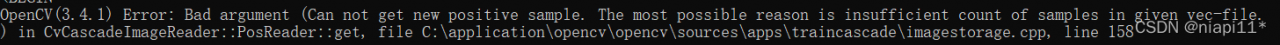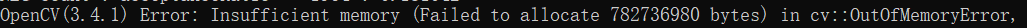# [Solved] OpenCV Train the class Error: Bad argument & Error: Insufficient memory

```OpenCV(3.4.1) Error: Bad argument (Can not get new positive sample. The most possible reason is insufficient count of samples in given vec-file.Solution: reduce the number of positive samples

opencv_traincascade.exe -data data_2 -vec positives.vec -bg bg.txt -numPos 350 -numNeg 1963 -mem 8192 -numStages 20 -w 20 -h 20

Here, you can reduce the numpos value

`OpenCV(3.4.1) Error: Insufficient memory (Failed to allocate 782736980 bytes) in cv::OutOfMemoryError, file C:\application\opencv\opencv\sources\modules\core\src\alloc.cpp, line 55`

Solution: increase memoryHere, increase the value of -mem and decrease the value of numPos

Summary: these two errors mainly lie in that the value of the number of positive samples for each level of training is too large. Numpos must be less than the total number of positive samples. Modifying MEM value only improves the running speed